# 1299 Is This Puzzle a Real Turkey?

Happy Thanksgiving, everyone!

Turkeys run but they cannot hide. They all will eventually end up on somebodies’ table. There doesn’t seem to be much of a mystery about that, but I’ve created a mystery level puzzle for today anyway. I promise it can be solved using logic and the basic facts in a 12 × 12 multiplication table.Print the puzzles or type the solution in this excel file: 12 factors 1289-1299

Now I’ll share some facts about the number 1299:

• 1299 is a composite number.
• Prime factorization: 1299 = 3 × 433
• The exponents in the prime factorization are 1 and 1. Adding one to each and multiplying we get (1 + 1)(1 + 1) = 2 × 2 = 4. Therefore 1299 has exactly 4 factors.
• Factors of 1299: 1, 3, 433, 1299
• Factor pairs: 1299 = 1 × 1299 or 3 × 433
• 1299 has no square factors that allow its square root to be simplified. √1299 ≈ 36.04164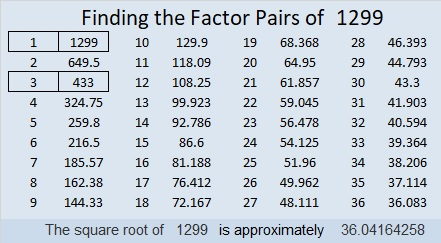1299 is the hypotenuse of a Pythagorean triple:
435-1224-1299 which is 3 times (145-408-433)

OEIS.org informs us that 8¹²⁹⁹ ≈ 1299 × 10¹¹⁷⁰. You can see it for yourself on a computer calculator!

# 966 Groan! Gotta Loosen My Belt

If you’ve overeaten this Thanksgiving day, you may be in too much pain to start working off all those extra calories. You may just want to loosen your belt and lie down somewhere while you groan about eating so much. Exercising your brain may help you alleviate some of that regret. This puzzle with its Pilgrim belt buckle could be just what you need. It’s a level 6 so it won’t be easy, but you will feel very accomplished if you can solve it.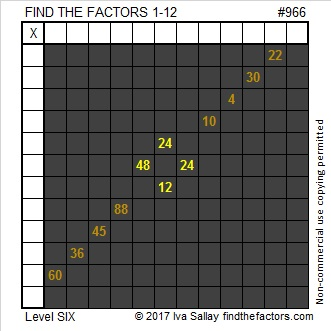Print the puzzles or type the solution in this excel file: 12 factors 959-967

Here’s a little information about the number 966:

It is the sum of eight consecutive prime numbers:
103 + 107 + 109 + 113 + 127 + 131 + 137 + 139 = 966

It is also the sum of two consecutive prime numbers:
467 + 479 = 966

966 is palindrome 686 in BASE 12 because 6(144) + 8(12) + 6(1) = 966

• 966 is a composite number.
• Prime factorization: 966 = 2 × 3 × 7 × 23
• The exponents in the prime factorization are 1, 1, 1, and 1. Adding one to each and multiplying we get (1 + 1)(1 + 1)(1 + 1)(1 + 1) = 2 × 2 × 2 × 2 = 16. Therefore 966 has exactly 16 factors.
• Factors of 966: 1, 2, 3, 6, 7, 14, 21, 23, 42, 46, 69, 138, 161, 322, 483, 966
• Factor pairs: 966 = 1 × 966, 2 × 483, 3 × 322, 6 × 161, 7 × 138, 14 × 69, 21 × 46, or 23 × 42
• 966 has no square factors that allow its square root to be simplified. √966 ≈ 31.08054# 965 Run, Turkey, Run!

Happy Thanksgiving, everyone!

I didn’t mean to make any Thanksgiving puzzles but after I created the puzzles this week, I could see some Thanksgiving-related pictures in the designs I had already made. This one is my favorite.

Run, Turkey, Run! For millions of turkeys today, it’s already too late.

Here is a little about the number 965:

965 is the sum of two squares two different ways:
26² + 17² = 965
31² + 2² = 965

So it is also the hypotenuse of FOUR Pythagorean triples, two of them primitives:
124-957-965, calculated from 2(31)(2), 31² – 2², 31² + 2²
387-884-965, calculated from 26² – 17², 2(26)(17), 26² + 17²
475-840-965 which is 5 times (95-168-193)
579-772-965 which is (3-4-5) times 193

• 965 is a composite number.
• Prime factorization: 965 = 5 × 193
• The exponents in the prime factorization are 1 and 1. Adding one to each and multiplying we get (1 + 1)(1 + 1) = 2 × 2 = 4. Therefore 965 has exactly 4 factors.
• Factors of 965: 1, 5, 193, 965
• Factor pairs: 965 = 1 × 965 or 5 × 193
• 965 has no square factors that allow its square root to be simplified. √965 ≈ 31.064449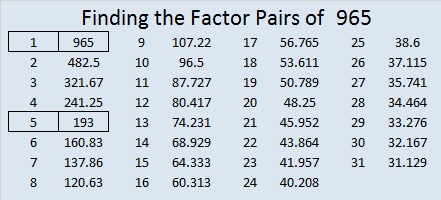# 964 Bow and Arrow

You can google lots of images of bows and arrows related to Thanksgiving. Perhaps that is because Indians from the Wampanoag Nation joined the Pilgrims in what we call the first Thanksgiving. I’m sure you know the story very well told from the white man’s point of view. It will be well worth your time to read The REAL Story of Thanksgiving as well. Everyone’s History Matters is also an excellent read.Print the puzzles or type the solution in this excel file: 12 factors 959-967

Now I’ll tell you a little about the number 964:

964 is the sum of the fourteen prime numbers from 41 to 101.

It is also the sum of four consecutive prime numbers:
233 + 239 + 241 + 251 = 964

30² + 8²  = 964 making it the hypotenuse of a Pythagorean triple:
480-836-964 which is 4 times (120-209-241)

964 is a palindrome in 2 bases and a repdigit in another.
1022201 in BASE 3
7A7 in BASE 11 (A is 10 in base 10)
444 in BASE 15

• 964 is a composite number.
• Prime factorization: 964 = 2 × 2 × 241, which can be written 964 = 2² × 241
• The exponents in the prime factorization are 2 and 1. Adding one to each and multiplying we get (2 + 1)(1 + 1) = 3 × 2  = 6. Therefore 964 has exactly 6 factors.
• Factors of 964: 1, 2, 4, 241, 482, 964
• Factor pairs: 964 = 1 × 964, 2 × 482, or 4 × 241
• Taking the factor pair with the largest square number factor, we get √964 = (√4)(√241) = 2√241 ≈ 31.048349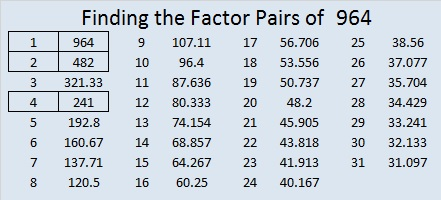# 962 Buckle Up, Pilgrim

Perhaps the most distinctive element of the pilgrims’ wardrobe is the buckle. We see it around the men’s waists, on their shoes, and on their hats. At least we do we if are looking at pilgrim costumes. It probably didn’t play as prominent a role on their actual clothes. Nevertheless, it is featured here on today’s puzzle. So buckle up and see where this puzzle takes you.Print the puzzles or type the solution in this excel file: 12 factors 959-967

Here are a few facts about the number 962:

It is the sum of two squares two different ways:
31² +  1² = 962
29² +  11² =  962

It is also the hypotenuse of four Pythagorean triples:
62-960-962, calculated from 2(31)(1), 31² –  1², 31² +  1²
312-910-962, which is 2 times (156-455-481)
370-888-962, which is 2 times (185-444-481)
638-720-962, calculated from 2(29)(11), 29² –  11², 29² +  11²

It looks interesting in a few other bases:
4242 BASE 6
282 BASE 20
101 BASE 31
QQ BASE 36 (Q is 26 in base 10)

• 962 is a composite number.
• Prime factorization: 962 = 2 × 13 × 37
• The exponents in the prime factorization are 1, 1, and 1. Adding one to each and multiplying we get (1 + 1)(1 + 1)(1 + 1) = 2 × 2 × 2 = 8. Therefore 962 has exactly 8 factors.
• Factors of 962: 1, 2, 13, 26, 37, 74, 481, 962
• Factor pairs: 962 = 1 × 962, 2 × 481, 13 × 74, or 26 × 37
• 962 has no square factors that allow its square root to be simplified. √962 ≈ 31.01612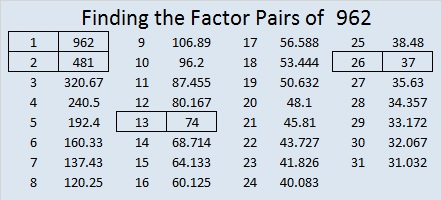# 692 Happy Thanksgiving!

• 692 is a composite number.
• Prime factorization: 692 = 2 x 2 x 173, which can be written 692 = (2^2) x 173
• The exponents in the prime factorization are 2 and 1. Adding one to each and multiplying we get (2 + 1)(1 + 1) = 3 x 2  = 6. Therefore 692 has exactly 6 factors.
• Factors of 692: 1, 2, 4, 173, 346, 692
• Factor pairs: 692 = 1 x 692, 2 x 346, or 4 x 173
• Taking the factor pair with the largest square number factor, we get √692 = (√4)(√173) = 2√173 ≈ 26.30589.Today is Thanksgiving in the United States. Regardless of where we live, there are reasons to be grateful today and every other day. Corrie ten Boom was grateful even when her circumstances were horrible. Here’s a Thanksgiving-themed puzzle to solve: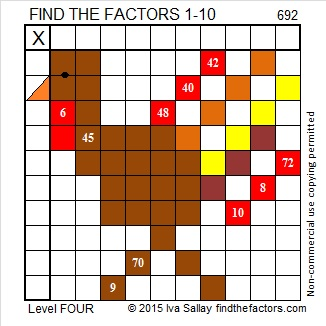Sometimes color in a puzzle is a distraction. Here’s the same puzzle minus the color:Print the puzzles or type the solution on this excel file: 10 Factors 2015-11-23

————————————————————————————

Here’s a little more about the number 692:

Because 173 is one of its factors, 692 is the hypotenuse of Pythagorean triple 208-660-692. What is the greatest common factor of those three numbers?

692 is a palindrome in 2 bases:

• 221122 BASE 3; note that 2(243) + 2(81) + 1(27) + 1(9) + 2(3) + 2(1) = 692
• 848 BASE 9; note that 8(81) + 4(9) + 8(1) = 692

————————————————————————————# 11 Counting Blessings

• 11 is a prime number.
• Prime factorization: 11 is prime.
• The exponent of prime number 11 is 1. Adding 1 to that exponent we get (1 + 1) = 2. Therefore 11 has exactly 2 factors.
• Factors of 11: 1, 11
• Factor pairs: 11 = 1 x 11
• 11 has no square factors that allow its square root to be simplified. √11 ≈ 3.31662.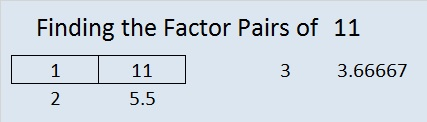How do we know that 11 is a prime number? If 11 were not a prime number, then it would be divisible by at least one prime number less than or equal to √11 ≈ 3.3. Since 11 cannot be divided evenly by 2 or 3, we know that 11 is a prime number.

Sometimes 11 is a clue in the FIND THE FACTORS 1 – 12 puzzles, and the factors are always 1 and 11.

I have more blessings than I could ever completely count. This is not the place where I will attempt to name them one by one, but I wonder: is the number of blessings that I or anyone else has finite or infinite? Even being able to ponder that question is a blessing. In the last few years in the United States, much of the gratitude part of Thanksgiving has gotten lost in commercialism. Therefore, for some people the number of blessings may be finite and easily measured by counting things. Some of those blessings may be more imaginary than real. Nevertheless, there are still people who can see the hand of God all around them. For them the number of blessings is infinite. Likewise those who rely on the Savior and His infinite atonement have an infinite number of blessings. As I count blessings, I find that some of them are prime, and some are a composite of several blessings working together. Some blessings are rather odd while others are shared evenly. I am grateful for many positive events in my life, but even negative experiences are blessings because they have helped me to grow.

The following blessings may seem trivial, but I am grateful that WordPress has given me a way to share the Find the Factors puzzles not only as jpg pictures, but also in an excel file.  The puzzles have been a blessing to me, and I want to show my gratitude by sharing them with other people. I am grateful for the blogs I follow. They challenge me, entertain me, and teach me so much. I am also thankful to everyone who has looked at my blog.

Click 12 Factors 2013-11-28 to see the same puzzles in excel.